Publication date: 08/13/2020

## Effect Details

The Effect Details report provides details, plots, and tests for individual effects. It consists of separate reports based on the emphasis that you select in the Fit Model launch window.

Effect Leverage emphasis: Each effect has its own report at the top of the Fit Least Squares report window to the right of the Whole Model report. In this case, the report includes a Leverage Plot for the effect.

Effect Screening or Minimal Report emphases: The Effect Details report is provided but is initially closed. Click the disclosure icon to show the report.

The initial content of the report is the Table of Least Squares Means. Depending on the nature of the effect, this table might not be appropriate, and the default report might initially show no content. However, certain red triangle options are available.

### Table of Effect Options

The red triangle menu next to an effect name provides the following options. For certain modeling types, some of these options might not be appropriate and are therefore not available.

LSMeans Table

Shows the statistics that are compared when effects are tested. See LSMeans Table.

This option is not enabled for continuous effects.

LSMeans Plot

Shows plots of least squares means for nominal and ordinal effects. If the effect is an interaction, this option displays the Least Squares Means Plot Options window. See LSMeans Plot.

LSMeans Contrast

Shows the Contrast Specification window, which enables you to specify and test contrasts to compare levels for nominal and ordinal effects and their interactions. See LSMeans Contrast.

LSMeans Student’s t

Shows tests and confidence intervals for pairwise comparisons of least squares means using Student’s t tests. See LSMeans Student’s t and LSMeans Tukey HSD.

Note: The significance level applies to individual comparisons and not to all comparisons collectively. The error rate for the collection of comparisons is greater than the error rate for individual tests.

LSMeans Tukey HSD

Shows tests and confidence intervals for pairwise comparisons of least squares means using the Tukey-Kramer HSD (Honestly Significant Difference) test (Tukey 1953; Kramer 1956). See LSMeans Student’s t and LSMeans Tukey HSD.

Note: The significance level applies to the collection of pairwise comparisons. The significance level is exact if the sample sizes are equal and conservative if the sample sizes differ (Hayter 1984).

LSMeans Dunnett

Shows tests and confidence intervals for pairwise comparisons against a control level that you specify. Also provides a plot of test results. See LSMeans Dunnett.

Test Slices

For each level of each column in the interaction, jointly tests pairwise comparisons among all the levels of the other classification columns in the interaction. See Test Slices.

Note: Available only for interactions involving nominal and ordinal effects.

Power Analysis

Shows the Power Details report, which enables you to analyze the power for the effect test. See Power Analysis.

### LSMeans Table

Least squares means are values predicted by the model for the levels of a categorical effect where the other model factors are set to neutral values. The neutral value for a continuous effect is defined to be its sample mean. The neutral value for a nominal effect that is not involved in the effect of interest is the average of the coefficients for that effect. The neutral value for an uninvolved ordinal effect is defined to be the first level of the effect in the value ordering.

Least squares means are also called adjusted means or population marginal means. Least squares means can differ from simple means when there are other effects in the model. In fact, it is common for the least squares means to be closer together than the sample means. This situation occurs because of the nature of the neutral values where these predictions are made.

Because least squares means are predictions at specific values of the other model factors, you can compare them. When effects are tested, comparisons are made using the least squares means. For more information about least squares means, see Least Squares Means across Nominal Factors in the Statistical Details section and Ordinal Least Squares Means.

For main effects, the Least Squares Means Table also includes the sample mean (Figure 3.10).

#### Example of a Least Squares Means Table

1. Select Help > Sample Data Library and open Big Class.jmp.

2. Select Analyze > Fit Model.

3. Select weight and click Y.

4. Select age, sex, and height and click Add.

5. From the Emphasis list, select Effect Screening.

6. Click Run.

7. The Effect Details report appears near the bottom of the Fit Least Squares report and is initially closed. Click the disclosure icon next to the Effect Details report title to show the report.

The Effect Details report, shown in Figure 3.10, shows reports for each of the three effects. Least Squares Means tables are given for age and sex, but not for the continuous effect height. Notice how the least squares means differ from the sample means.

Figure 3.10 Least Squares Mean Table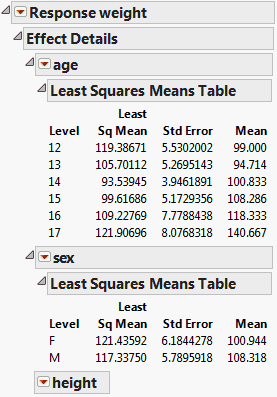The Least Squares Means report contains the following columns:

Level

The categorical levels or combination of levels.

Least Sq Mean

An estimate of the least squares mean for each level.

Estimability

(Appears only when a least squares mean is not estimable.) A warning if a least squares mean is not estimable.

Std Error

Shows the standard error of the least squares mean for each level.

Lower 95%

Shows the lower 95% confidence limit for the least squares mean. This column appears only if you have the Regression Reports > Show All Confidence Intervals option selected or if you right-click in the report and select Columns > Lower 95%.

Upper 95%

Shows the upper 95% confidence limit for the least squares mean. This column appears only if you have the Regression Reports > Show All Confidence Intervals option selected or if you right-click in the report and select Columns > Upper 95%.

Mean

Shows the response sample mean for the given level. This mean differs from the least squares mean if the values for other effects in the model do not balance out across this effect.

### LSMeans Plot

The LSMeans Plot option produces a Least Squares Means Plot for nominal and ordinal main effects and their interactions. If the effect is an interaction, this option displays the Least Squares Means Plot Options window. See Least Squares Means Plot Options.

The Least Squares Means Plot red triangle menu contains the following options:

Show Confidence Limits

Shows or hides confidence limits for each estimate in the plot.

Show Connected Points

Shows or hides one or more lines that connect the least squares means for each level in the plot.

Remove

Removes the Least Squares Means Plot report for the specified effect.

#### Least Squares Means Plot Options

When you select the LSMeans Plot option from the red triangle menu of an interaction effect, the Least Squares Means Plot Options window appears.

Figure 3.11 Least Squares Means Plot Options Window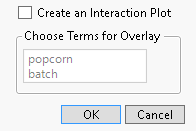If you click OK without selecting anything in the window, one LSMeans Plot appears. The horizontal axis of the plot consists of the levels of the factors nested to obtain a separate effect for each combination. To create an interaction plot, select the box next to Create an Interaction Plot. The Choose Terms for Overlay option enables you to select which effect is displayed as the overlay variable in the interaction plot.

For a three-way interaction term, a second panel of options appears after you choose an overlay variable for the interaction plot. If you click OK without selecting anything in the second panel, one interaction plot appears. Alternatively, use the second panel of options to create separate interaction plots for each level of the effect that you select under Choose Terms for Separate Plots.

Figure 3.12 Least Squares Means Tables and Plots for Two Effects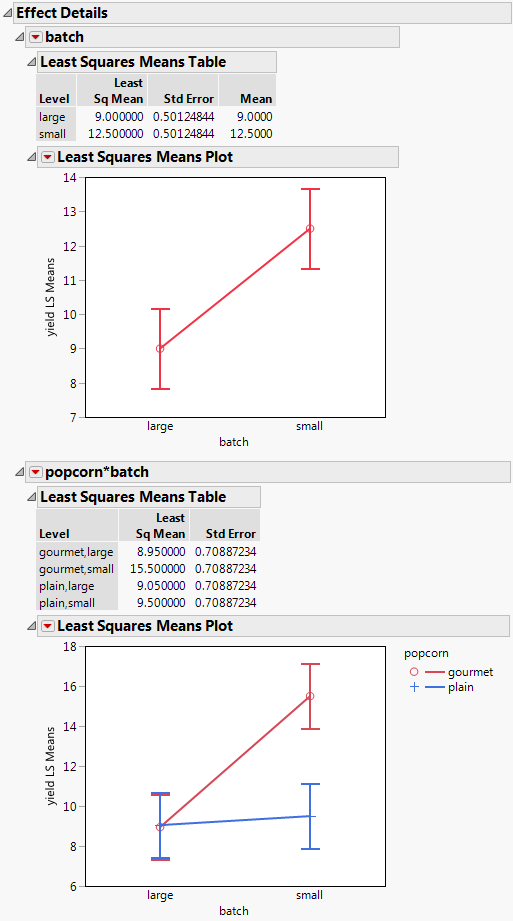#### Example of an LS Means Plot

To create the report in Figure 3.12, follow these steps:

1. Select Help > Sample Data Library and open Popcorn.jmp.

2. Select Analyze > Fit Model.

3. Select yield and click Y.

4. Select popcorn, oil amt, and batch and click Macros > Full Factorial. Note that the Emphasis changes to Effect Screening.

5. Click Run.

6. Click the Effect Details disclosure icon to show the details for the seven model effects.

7. Click the batch red triangle and select LSMeans Plot.

8. Click the popcorn*batch red triangle and select LSMeans Plot. The Least Squares Means Plot Options window appears.

9. In the Least Squares Means Plot Options window, click the box next to Create an Interaction Plot.

10. Under Choose Terms for Overlay, select popcorn.

11. Click OK.

12. To transpose the factors in the plot for popcorn*batch, repeat step 8 and step 9.

13. Under the Choose Terms for Overlay, select batch and click OK.

Figure 3.13 LSMeans Plot for Interaction with Factors Transposed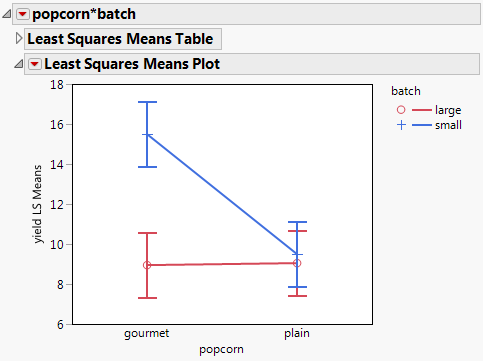Figure 3.13 shows the popcorn*batch interaction plot with the factors transposed. Compare it with the plot in Figure 3.12. These plots depict the same information but, depending on your interest, one might be more intuitive than the other.

### LSMeans Contrast

A contrast is a linear combination of parameter values. In the Contrast Specification window, you can specify multiple contrasts and jointly test whether they are zero (Figure 3.14).

JMP builds contrasts in terms of the least squares means of the effect. Each column of the contrast is normalized to have sum zero and so that the sum of the absolute values equals two. If a contrast involves a covariate, you can specify the value of the covariate at which to test the contrast.

The Contrast Specification box shows the name of the effect and the names of the levels in the effect. The contrast values are initially set to zero and appear next to cells containing + and - signs. Click these buttons to compare levels.

Each time you click the + or - button, the contrast coefficients are normalized to make their sum zero and their absolute sum equal to two, if possible. To compare additional levels, click the New Column button. A new column appears in which you define a new contrast. After you are finished, click Done. The Contrast report appears (Figure 3.15). The overall test is a joint F test for all contrasts.

Note: If you attempt to specify more than the maximum number of contrasts possible, the test automatically evaluates.

The Contrast report provides the following details about the joint F test:

SS

The sum of squares for the joint test.

NumDF

The numerator degrees of freedom.

DenDF

The denominator degrees of freedom.

F Ratio

The ratio of SS divided by NumDF divided by the mean square error.

Prob > F

The p-value for the significance test.

#### Test Detail Report

The Test Detail report (Figure 3.15) shows a column for each contrast that you tested. For each contrast, the report gives its estimated value, its standard error, a t ratio for a test of that single contrast, the corresponding p-value, and its sum of squares.

#### Parameter Function Report

The Parameter Function report (Figure 3.15) shows the contrasts that you specified expressed as linear combinations of the terms of the model.

#### Example of LSMeans Contrast

To illustrate the LSMeans Contrast option, form a contrast that compares the first two age levels with the next two levels.

Follow these steps to create the report shown in Figure 3.14.

1. Select Help > Sample Data Library and open Big Class.jmp.

2. Select Analyze > Fit Model.

3. Select weight and click Y.

4. Select age, sex, and height, and click Add.

5. Select age in the Select Columns list, select height in the Construct Model Effects list, and click Cross.

6. Click Run.

The Fit Least Squares report appears.

7. Click the age red triangle and select LSMeans Contrast.

Figure 3.14 LSMeans Contrast Specification for age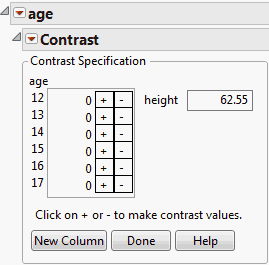8. Click “+” for the ages 12 and 13.

9. Click “-” for ages 14 and 15.

This contrast tests whether the mean weights differ for the two age groups, based on predicted values at a height of 62.55.

10. Note that there is a text box next to the continuous effect height. The default value is the mean of the continuous effect.

11. Click Done.

12. Open the Test Detail and Parameter Function reports.

The Contrast report is shown in Figure 3.15. The test for the contrast is significant at the 0.05 level. You conclude that the predicted weight for age 12 and 13 children differs statistically from the predicted weight for age 14 and 15 children at the mean height of 62.55.

Figure 3.15 LSMeans Contrast Report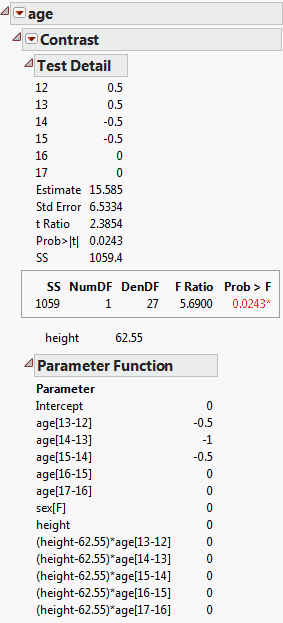### LSMeans Student’s t and LSMeans Tukey HSD

The LSMeans Student’s t and LSMeans Tukey HSD (honestly significant difference) options test pairwise comparisons of model effects.

The LSMeans Student’s t option is based on the usual independent samples, equal variance t test. Each comparison is based on the specified significance level. The overall error rate resulting from conducting multiple comparisons exceeds that specified significance level.

The LSMeans Tukey HSD option conducts Tukey HSD tests. For these comparisons, the significance level applies to the entire collection of pairwise comparisons. For this reason, confidence intervals for LS Means Tukey HSD are wider than those for LSMeans Student’s t. The significance level is exact if the sample sizes are equal and conservative if the sample sizes differ (Hayter 1984).

Figure 3.16 shows the LSMeans Tukey report for the effect age in the Big Class.jmp sample data table. (To obtain this report, run the Fit Model data table script, click the age red triangle, and select LS Means Tukey HSD.) By default, the report shows the Crosstab Report and the Connecting Letters Report.

Figure 3.16 LSMeans Tukey HSD Report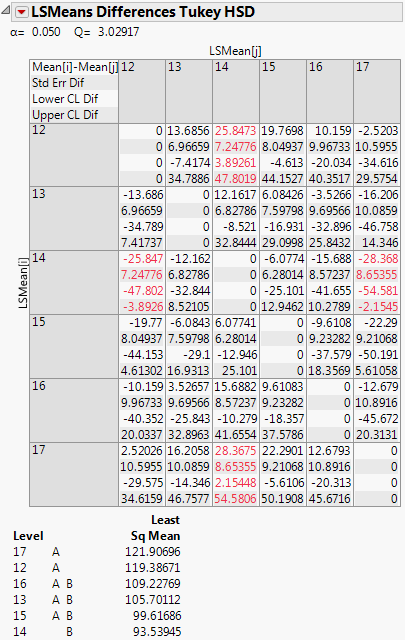#### The Crosstab Report

Both options display a matrix, called the Crosstab Report, where each cell contains the difference in means, the standard error of the difference, and lower and upper confidence limits. The significance level and corresponding critical value are given above the matrix. The default significance level is 0.05, but you can specify a different significance level in the Fit Model launch window. Cells that correspond to pairs of means that differ statistically are shown in red.

#### The Connecting Letters Report

A Connecting Letters Report appears by default beneath the Crosstab matrix. Levels that share, or are connected by, the same letter do not differ statistically. Levels that are not connected by a common letter do differ statistically.

In Figure 3.16, levels 17, 12, 16, 13, and 15 are connected by the letter A. The connection indicates that these levels do not differ at the 0.05 significance level. Also, levels 16, 13, 15, and 14 are connected by the letter B, indicating that they do not differ statistically. However, ages 17 and 14, and ages 12 and 14, are not connected by a common letter, indicating that these two pairs of levels are statistically different.

Tip: Right-click in the connecting letters report and select Columns to add columns containing connecting letters (Letters), standard errors (Std Error), and confidence interval limits (Lower X% and Upper X%). In the Letters column, the connecting letters are concatenated into a single column. The significance and confidence levels are determined by the significance level that you specify in the Fit Model launch window using the Set Alpha Option.

#### LSMeans Student’s t and LSMeans Tukey HSD Options

The red triangle options that appear in each report window show or hide optional reports. All of the options below are available for LSMeans Student’s t. The first four options are available for LSMeans Tukey HSD. For both LSMeans Student’s t and LSMeans Tukey HSD, the Crosstab Report and the Connecting Letters Report are shown by default.

Crosstab Report

Shows a two-way table that provides, for each pair of levels, the difference in means, the standard error of the difference, and confidence limits for the difference. The contents of cells containing significant differences are highlighted in red.

Connecting Letters Report

Illustrates significant and non-significant comparisons with connecting letters. Levels not connected by the same letter are significantly different. Levels connected by the same letter are not significantly different.

Save Connecting Letters Table

Creates a data table whose columns give the levels of the effect, the connecting letters, the least squares means, their standard errors, and confidence intervals. The table contains a script called Bar Chart that produces a colored bar chart of the least squares means with their confidence intervals superimposed. The levels are arranged in decreasing order of least squares means.

Figure 3.17 shows the bar chart for an example based on Big Class.jmp. Run the Fit Model data table script, click the age red triangle, and select LSMeans Tukey HSD. Select Save Connecting Letters Table from the LSMeans Differences Tukey HSD report. Run the Bar Chart script in the data table that appears.

Ordered Differences Report

Ranks the differences from largest to smallest, giving standard errors, confidence limits, and p-values. Also plots the differences on a bar chart with overlaid confidence intervals.

Detailed Comparisons

Gives individual detailed reports for each comparison. For a given comparison, the report shows the estimated difference, standard error, confidence interval, t ratio, degrees of freedom, and p-values for one- and two-sided tests. Also shown is a plot of the t distribution, which illustrates the significance test for the comparison. The area of the shaded portion is the p-value for a two-sided test.

Figure 3.17 Bar Chart from LSMeans Differences HSD Connecting Letters Table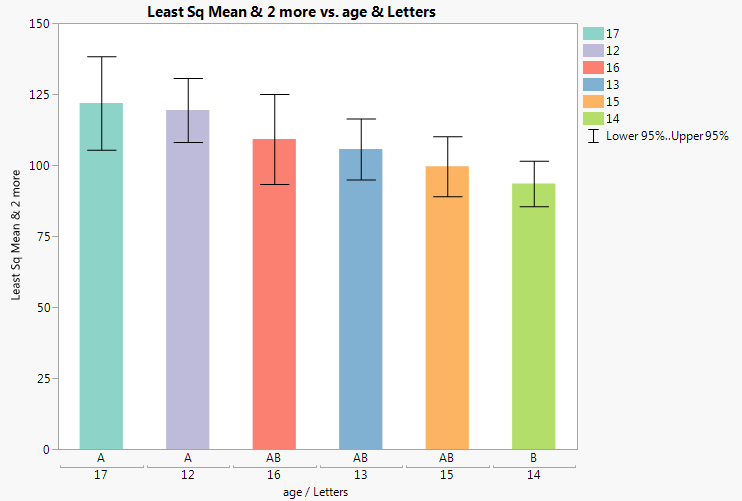### LSMeans Dunnett

Dunnett’s test (Dunnett 1955) compares a set of means against the mean of a control group. The error rate applies to the collection of pairwise comparisons. The LSMeans Dunnett option conducts Dunnett’s test for the levels of the given effect. Hsu’s factor analytical approximation is used for the calculation of p-values and confidence intervals (Hsu 1992).

When you select LSMeans Dunnett, you are prompted to enter a control level for the effect. The LS Means Differences Hsu-Dunnett report shows the significance level, the value of the test statistic (Q), and the control level.

A report for the LSMeans Dunnett option for effect treatment in the Cholesterol.jmp sample data table is shown in Figure 3.18. Here, the response is June PM and the level of treatment called Control is specified as the control level.

Figure 3.18 LSMeans Dunnett Report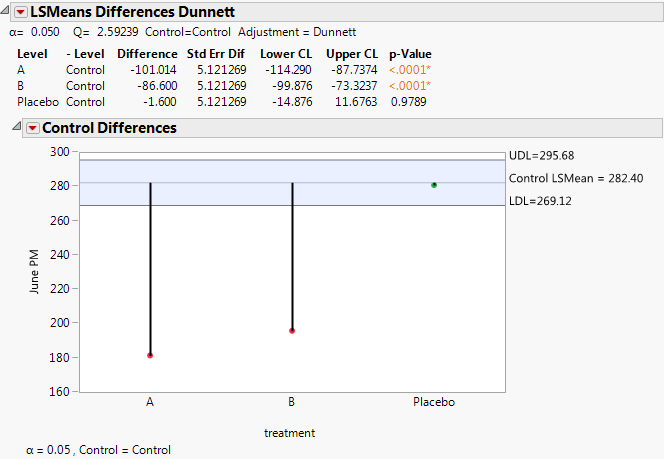The report has two options:

Control Differences Report

The Control Differences report is shown by default. For each level of the effect, a table shows the following information: the level being compared to the control level, the estimated difference, the standard error of the difference, a confidence interval, and the p-value for the comparison.

Control Differences Chart

For each level other than the control, a point shows the difference between the LS Mean for that level and the LS Mean for the control level. Upper and lower decision limits (UDL, LDL) are plotted. The report has a Show Summary Report option and Display options. The Show Summary Report option gives the plot detail. The Display options enable you to modify the plot appearance.

### Test Slices

The Test Slices option is enabled for interaction effects composed of nominal or ordinal columns. For each level of each nominal or ordinal column in the interaction, this option produces a report that jointly tests all pairwise comparisons of settings involving that level. The test is effectively a test of differences within the specified “slice” of the interaction.

Suppose that you are interested in an A*B*C interaction, where one of the levels of A is “Small”. The Test Slice report for the slice A = Small jointly tests all pairwise comparisons of the B*C levels when A = Small. It enables you to detect differences in levels within an interaction.

The Test Slice reports follow the same format as do the LSMeans Contrast reports. See LSMeans Contrast.

### Power Analysis

Opens the Power Details window, where you can enter information to obtain retrospective or prospective details for the F test of a specific effect.

Note: To ensure that your study includes sufficiently many observations to detect the required differences, use information about power when you design your experiment. Such an analysis is called a prospective power analysis. Consider using the DOE platform to design your study. Both DOE > Sample Size and Power and DOE > Evaluate Design are useful for prospective power analysis. For an example of a prospective power analysis using standard least squares, see Prospective Power Analysis.

Figure 3.19 shows an example of the Power Details window for the Big Class.jmp sample data table. Using the Power Details window, you can explore power for values of alpha (α), sigma (σ), delta (δ), and Number (study size). Enter a single value (From only), two values (From and To), or the start (From), stop (To), and increment (By) for a sequence of values. Power calculations are reported for all possible combinations of the values that you specify.

Figure 3.19 Power Details Window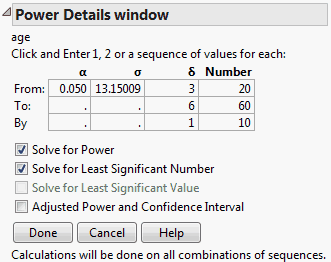See Power Analysis.

The Power Details window report contains the following columns and options:

Alpha (α)

The significance level of the test. This value is between 0 and 1, and is often 0.05, 0.01, or 0.10. The initial value for Alpha, shown in the first row, is 0.05, unless you have selected Set Alpha Level and set a different value in the Fit Model launch window.

Sigma (σ)

An estimate of the residual error in the model. The initial value shown in the first row, provided for guidance, is the RMSE (the square root of the mean square error).

Delta (δ)

The effect size of interest. See Effect Size. The initial value, shown in the first row, is the square root of the sum of squares for the hypothesis divided by the square root of the number of observations in the study (that is,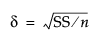).

Number (n)

The sample size. The initial value, shown in the first row, is the number of observations in the current study.

Solve for Power

Solves for the power as a function of α, σ, δ, and n. The power is the probability of detecting a difference of size δ by seeing a test result that is significant at level α, for the specified σ and n. See Computations for the Power in the Statistical Details section.

Solve for Least Significant Number

Solves for the smallest number of observations required to obtain a test result that is significant at level α, for the specified δ and σ. See Computations for the LSN in the Statistical Details section.

Solve for Least Significant Value

Solves for the smallest positive value of a parameter or linear function of the parameters that produces a p-value of α. The least significant value is a function of α, σ, and n. This option is available only for one-degree-of-freedom tests. See Computations for the LSV in the Statistical Details section.